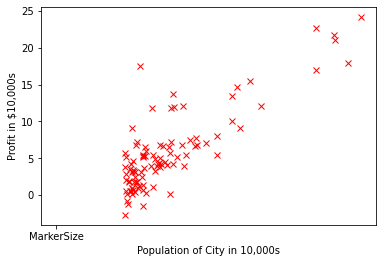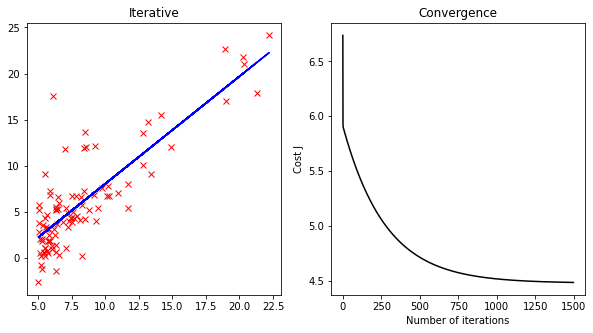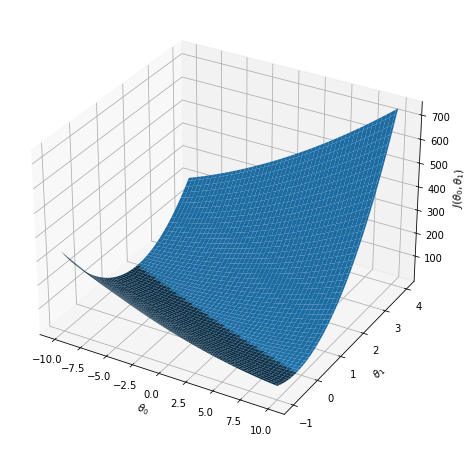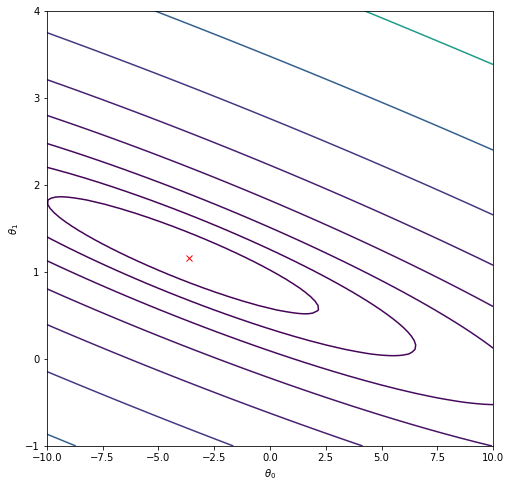In this exercise, I implemented in MATLAB and Python the linear regression model for one and multiple variables and got to see it work on data. The code is available for MATLAB and Python; however, I will be commenting only on Python code.

Key Takeaways:

1. Linear Regression with One and Multiple variables
2. Plotting the data
4. Cost function
5. Feature Normalization
6. Normal Equation

### Introduction

Throughout the exercise, we will be using the script linearRegression.py. This script sets up the dataset for the problems and makes calls to other functions. For this programming exercise, we completed it implementing the linear regression with one variable and with multiple variables. We can check below the initial import and setup for the Python code.

from matplotlib import pyplot as plt
import os
import numpy as np
from sklearn import linear_model

from mapFeature import mapFeature
from featureNormalize import featureNormalize
from costFunction import costFunction
from plotData import plotConvergence, plotIterative, plotNormalEqn, plotSklearn
from normalEqn import normalEqn

# Gradient descent settings

order = 1 # Doesn't work for more features
lamb  = 0 # Regularization
normalize = False


### Linear Regression with one variable

In this part of this exercise, we will implement linear regression with one variable to predict profits for a food truck. Suppose you are the CEO of a restaurant franchise and are considering different cities for opening a new outlet. The chain already has trucks in various cities and you have data for profits and populations from the cities.

You would like to use this data to help you select which city to expand to next. The file ex1data1.txt contains the dataset for our linear regression problem. The first column is the population of a city and the second column is the profit of a food truck in that city. A negative value for profit indicates a loss.

The linearRegression.py script has already been set up to load this data.

#### Plotting the Data

Before starting on any task, it is often useful to understand the data by visualizing it. For this dataset, we can use a scatter plot to visualize the data, since it has only two properties to plot (profit and population). (Many other problems that we will encounter in real life are multi-dimensional and can’t be plotted on a 2-d plot).

In linearRegression.py, the dataset is loaded from the data file into the variables X and y:

os.system('clear')  # For Linux/OS X - Clear the console
data = np.loadtxt("ex1data1.txt", dtype='f', delimiter=',') # read comma separated data

x = data[:,0].reshape(-1,1)
X = np.copy(x)
y = data[:,1].reshape(-1,1)
m = len(y) # number of training examples


Next, the script calls the plotData.py function to create a scatter plot of the data.

plt.plot(x, y, 'rx', 'MarkerSize', 10); # Plot the data
plt.ylabel('Profit in $10,000s'); # Set the y-axis label plt.xlabel('Population of City in 10,000s'); # Set the x-axis labelBelow you can check the plotData.py code. from matplotlib import pyplot as plt def plotIterative(x,y,h): # Plotting Linear Regression plt.subplot(121) plt.title('Iterative') plt.plot(x, y, 'rx') plt.plot(x, h,'b') def plotConvergence(J_history): # Plotting Convergence plt.subplot(122) plt.title('Convergence') plt.xlabel('Number of iterations') plt.ylabel('Cost J') plt.plot(range(len(J_history)), J_history,'k') def plotNormalEqn(x,y,h): # Plotting Linear Regression with Normal Equation plt.subplot(121) plt.title('Normal Equation') plt.plot(x, y, 'rx') plt.plot(x, h, 'g') def plotSklearn(x,y,h): # Plotting Linear Regression with Scikit-learn plt.subplot(122) plt.title('Sklearn') plt.plot(x, y,'rx') plt.plot(x, h.predict(x),'k')  #### Gradient descent In this part, we will fit the linear regression parameters $$\theta$$ to our dataset using gradient descent. The objective of linear regression is to minimize the cost function. $J(\theta)=\frac{1}{2m}\sum_{i=1}^{m}(h_{\theta}(x^{(i)})-y^{(i)})^2$ where the hypothesis $$h_{\theta}(x)$$ is given by the linear model $h_{\theta}(x)=\theta^Tx=\theta_0+\theta_1x_1$ The parameters of the model are the $$\theta_j$$ values. These are the values we will adjust to minimize cost $$J(\theta)$$. One way to do this is to use the batch gradient descent algorithm. In batch gradient descent, each iteration performs the update $\theta_j=\theta_j-\alpha\frac{1}{m}\sum_{i=1}^{m}(h_\theta(x^{(i)}-y^{(i)})x_j^{(i)})\quad(simultaneously\:update\:\theta_j\:for\: all\:j)$ With each step of gradient descent, the parameters $$\theta_j$$ come closer to the optimal values that will achieve the lowest cost $$J(\theta)$$. In linearRegression.py, we have set up the data for linear regression. In the following lines, we add another dimension to our data to accommodate the $$\theta_0$$ intercept term. We also initialize the initial parameters to 0 and the learning rate alpha to 0.01. # Add intercept term to X X = mapFeature(X, order = 1) theta = np.zeros((X.shape,1)) # Start t0 and t1 (Parameters) iterations = 1500; # 1500: 1 feature, 400: 2 features alpha = 0.01;  Follow the mapFeature function: import numpy as np def mapFeature(X, order): # one or two features out = [] try: for i in range(order+1): for j in range(i+1): out = np.concatenate((out, (X[:,0]**(i-j))*(X[:,1]**j))) out = np.reshape(np.ravel(out), (-1,int(out.shape/X[:,0].shape)), 'F') except Exception: for i in range(order+1): out = np.concatenate((out, (X[:,0]**i))) out = np.reshape(np.ravel(out), (-1,int(out.shape/X[:,0].shape)), 'F') return out  As we perform gradient descent to learn minimize the cost function $$J(\theta)$$, it is helpful to monitor the convergence by computing the cost. Here, we implement a function to calculate $$J(\theta)$$ so we can check the convergence of our gradient descent implementation. The next task is to create the code to computer the cost function costFunction.py, which is a function that computes $$J(\theta)$$. As we are doing this, remember that the variables X and y are not scalar values, but matrices whose rows represent the examples from the training set. import numpy as np def costFunction(X, y, theta, lamb): m = len(y) h = np.dot(X,theta) # J = (1/(2*m)) * sum((h - y)**2 + lamb*theta**2) J = (1/(2*m)) * (np.dot((h-y).T, (h-y)) + lamb*sum(theta[1:]**2)) return J  Once we have completed the function, the next step in linearRegression.py will run costFunction.py once using $$\theta$$ initialized to zeros, and we will see the cost printed to the screen. You should expect to see a cost of 32.07. print('Testing the Cost Function...\n') theta = np.zeros((X.shape,1)) # Start t0 and t1 (Parameters) J = costFunction(X, y, theta, lamb); print('With theta = [0 ; 0]\n\nCost computed = %f\n' % J); cost = lambda theta,X,y: (1/(2*len(y))) * sum((np.dot(X,theta) - y)**2) print('Expected cost value (approx) %f\n' % cost(theta,X,y))  Testing the Cost Function... With theta = [0 ; 0] Cost computed = 32.072734 Expected cost value (approx) 32.072734  Next, we will implement gradient descent in the file gradientDescent.py. Keep in mind that the cost $$J(\theta)$$ is parameterized by the vector $$\theta$$, not X and y. That is, we minimize the value of $$J(\theta)$$ by changing the values of the vector $$\theta$$, not by changing X or y. A good way to verify that gradient descent is working correctly is to look at the value of $$J(\theta)$$ and check that it is decreasing with each step. The code for gradientDescent.py calls costFunction.py on every iteration and prints the cost. The value of $$J(\theta)$$ should never increase, and should converge to a steady value by the end of the algorithm. Check below the code for gradientDescent.py. import numpy as np from costFunction import costFunction def gradientDescent(X, y, theta, alpha, num_iters, lamb): # alpha: Learning Rate, inter: Number of interactions ''' Setup the Parameters ''' m = len(y) J_history = np.zeros(num_iters).reshape(-1,1) for i in range(num_iters): # Number of interactions h = np.dot(X,theta) # Hypothesis Function ''' Gradient Descent applied to the Cost Function ''' # theta0 that doesn't need regularization t0 = theta * (1 - (alpha*0)/m) - (alpha/m) * np.dot(X.T, (h - y)) theta = theta * (1 - (alpha*lamb)/m) - (alpha/m) * np.dot(X.T, (h - y)) theta = t0 J_history[i] = costFunction(X, y, theta, lamb) return theta, J_history  After we finish it, linearRegression.py will use the final parameters to plot the linear fit. The result should look something like Figure below, where you can also check the convergence curve:The final values for $$\theta$$ will also be used to make predictions on profits in areas of 35,000 and 70,000 people. Note the way that the following lines in linearRegression.py uses matrix multiplication, rather than explicit summation or looping, to calculate the predictions. This is an example of code vectorization. xx = np.array([1, 70000]) predict1 = np.dot(theta1.T, xx) predict2 = np.dot(theta2.T, xx) predict3 = np.dot(theta3.T, xx) print('\npredict1: %f \npredict2: %f \npredict3: %f' % (predict1, predict2, predict3))  predict1: 81641.737254 predict2: 83508.462590 predict3: 83508.462885  #### Visualizing $$J(\theta)$$ To understand the cost function $$J(\theta)$$ better, we will now plot the cost over a 2-dimensional grid of $$\theta_0$$ and $$\theta_1$$ values. In the next step of linearRegression.py, there is code set up to calculate $$J(\theta)$$ over a grid of values using the costFuncion.py. os.system('clear') # For Linux/OS X print(' Visualization J(theta_0, theta_1) ... ') ''' Part 3: Visualization J(theta_0, theta_1) ''' # Grid over which we will calculate J fig = plt.figure(figsize=(8,8)) ax = fig.add_subplot(111, projection='3d') theta0_vals = np.linspace(-10, 10, 100) theta1_vals = np.linspace(-1, 4, 100) theta0_valsX, theta1_valsY = np.meshgrid(theta0_vals, theta1_vals) thetas = np.array((np.ravel(theta0_valsX), np.ravel(theta1_valsY))).T.reshape(-1,2,1) J_valsZ = np.array([costFunction(X,y,t,lamb) for t in thetas]) J_valsZ = J_valsZ.reshape(theta0_valsX.shape) ax.plot_surface(theta0_valsX, theta1_valsY, J_valsZ) ax.set_xlabel(r'$\theta_0$') ax.set_ylabel(r'$\theta_1$') ax.set_zlabel(r'$J(\theta_0, \theta_1)$') plt.show() plt.pause(0.01) input('\n\n\n\nPRESS ENTER...\n') os.system('clear') # For Linux/OS X print(' Visualization J(theta_0, theta_1) contour ... ') # Contour plot # Plot J_vals as 15 contours spaced logarithmically between 0.01 and 100 fig = plt.figure(figsize=(8,8)) plt.contour(theta0_vals, theta1_vals, J_valsZ, np.logspace(-2, 3, 20)) plt.xlabel(r'$\theta_0$') plt.ylabel(r'$\theta_1\$')
plt.plot(theta1, theta1, 'rx')


After these lines are executed, you will have a 2-D array of $$J(\theta)$$ values. The script linearRegression.py will then use these values to produce surface and contour plots of $$J(\theta)$$ using the surf and contour commands. The plots should look something like Figures below.The purpose of these graphs is to show how $$J(\theta)$$ varies with changes in $$\theta_0$$ and $$\theta_1$$. The cost function $$J(\theta)$$ is bowl-shaped and has a global mininum. (This is easier to see in the contour plot than in the 3D surface plot). This minimum is the optimal point for $$\theta_0$$ and $$\theta_1$$, and each step of gradient descent moves closer to this point.

### Linear regression with multiple variables

In this part, we will implement linear regression with multiple variables to predict the prices of houses. Suppose you are selling your house and you want to know what a good market price would be. One way to do this is to first collect information on recent houses sold and make a model of housing prices.

The file ex1data2.txt contains a training set of housing prices in Portland, Oregon. The first column is the size of the house (in square feet), the second column is the number of bedrooms, and the third column is the price of the house.